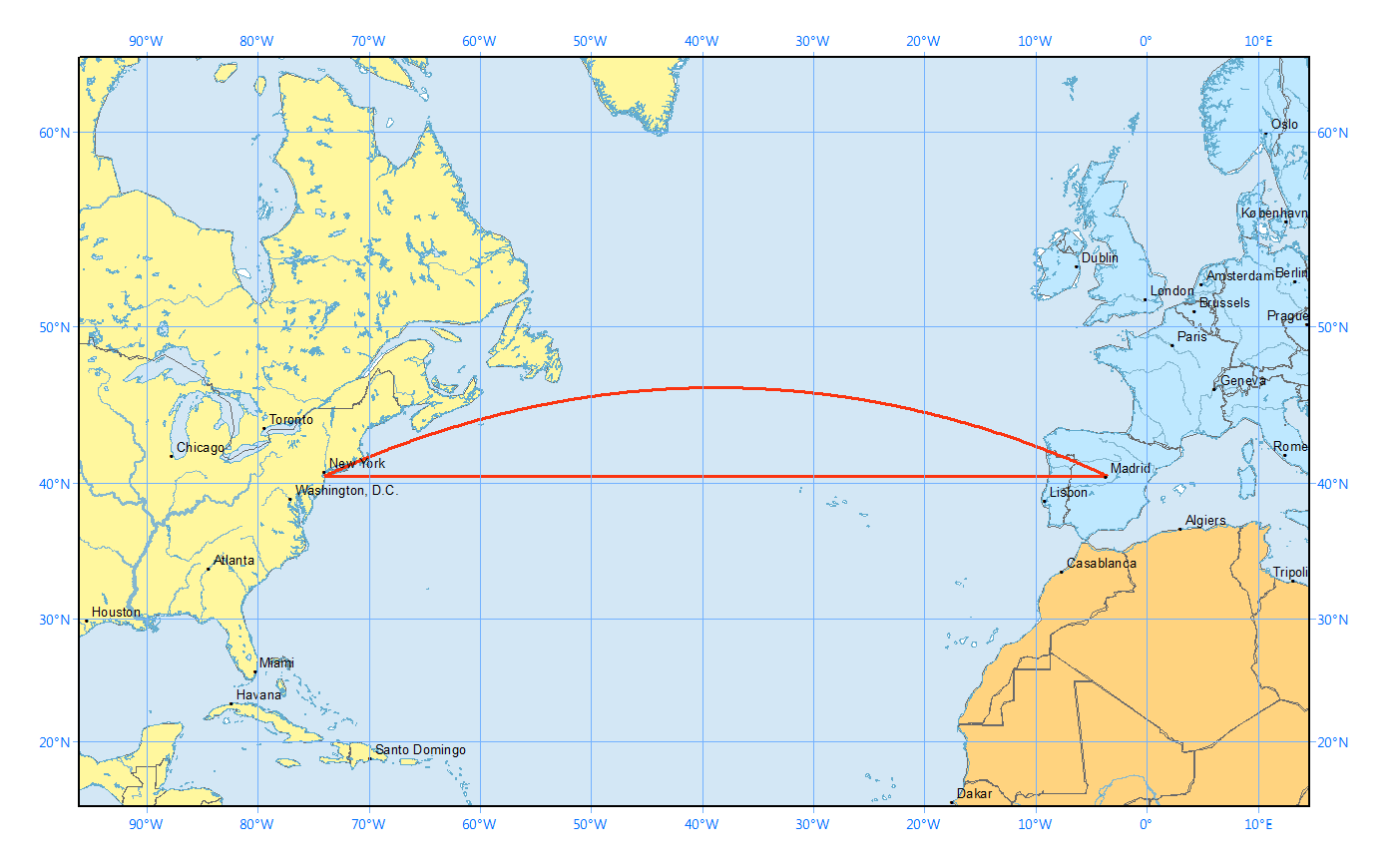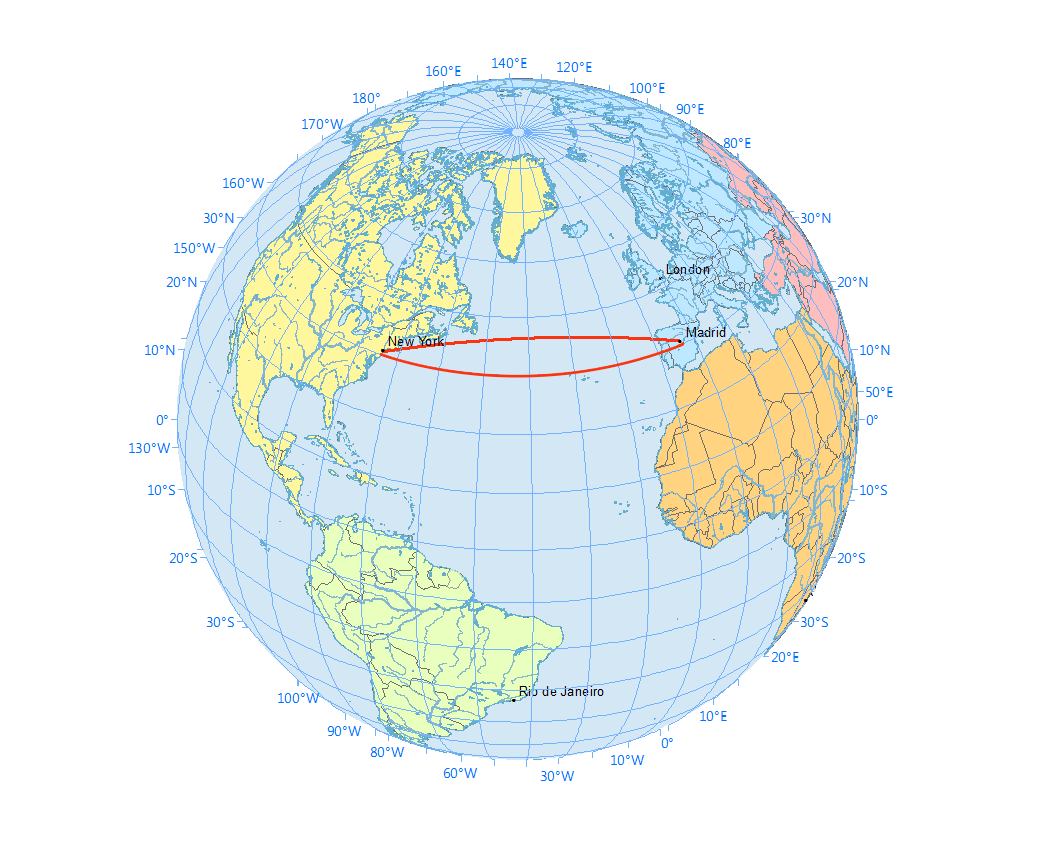Welcome to Project Lovelace! We're still in early development so there are still tons of bugs to find and improvements to make. If you have any suggestions, complaints, or comments please let us know on Discourse, Discord, or GitHub!

# Flight paths

## You will learn about: calculating distances on a sphere.

If you ever flew in a plane, you probably realized that the plane took a curved path instead of a straight path (if you were looking at a flat map). This is because on a sphere, the shortest distance between two points is along a great circle. This is more obvious when you plot the straight and curved paths on a flat map (which uses the Mercator projection) and on a sphere.On a flat map (Mercator projection) the shortest path from New York to Madrid seems to be along a constant latitude, but the Mercator projection inflates areas away from the equator. As a result, the curved path is actually shorter!Plotting the trajectories on a sphere makes it clearer that the curved path is actually shorter.

You can calculate the length of the curved path using the haversine formula. Between two points on the Earth $(\phi_1, \lambda_1)$ and $(\phi_2, \lambda_2)$ where $\phi$ is latitude and $\lambda$ is longitude, the shortest distance is $$2R \arcsin \sqrt{ \sin^2 \left( \frac{\phi_2 - \phi_1}{2} \right) + \cos\phi_1 \cos\phi_2 \sin^2 \left( \frac{\lambda_2 - \lambda_1}{2} \right)}$$ where $R$ = 6372.1 km is the radius of the Earth.

Input: (Latitude, longitude) coordinates of two points. Latitudes go from -90 to +90. Longitudes go from -180 to +180. Remember to convert your angles from degrees to radians before using the sine and cosine functions!

Output: The distance between the points in kilometers (km).

Example

Input point 1 (latitude, longitude) : 46.283, 86.667 Input point 2 (latitude, longitude) : -48.877, -123.393 Output distance: 17760.054
 Difficulty haversine_distance(lat1, lon1, lat2, lon2)

You must be logged in to view your submissions.

### Notes

• In this problem we use the haversine formula which calculates the great-circle distance assuming the Earth is a perfect sphere so it can be off by up to 0.5%. Vincenty's formulae account for the oblate spheroid shape of the Earth and are much more accurate, being off only by <0.5 mm.

Let us know what you think about this problem! Was it too hard? Difficult to understand? Also feel free to discuss the problem, ask questions, and post cool stuff on Discourse. You should be able see a discussion thread below. Would be nice if you don't post solutions in there but if you do then please organize and document your code well so others can learn from it.